Easily rotate Ellipse when Drawing on a graphHow hard would it be to enable easily rotating Ellipse objects created with the cmd+T Drawing Tools ?

Currently, user can intuitively re-size the ellipse but not rotate it. Perhaps we could use shift+mouse to enable rotation ? Or add a rotate "handle"   similar to what is done e.g. in powerpoint as shown below: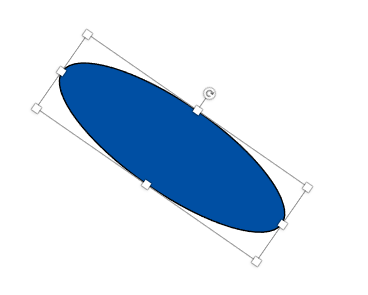Perhaps others have already done something similar ?

Ideally, this would work on Igor 8 as well, not just 9 ...

This works in Igor 9 already, but it is limited to ellipses in isotropic coordinate systems. That means that you can easily rotate an ellipse in a control panel or in a graph when using absolute coordinates.

In non-isotropic coordinate systems (anything that isn't absolute coordinates) you get weird effects as the ellipse dimensions rotate into different orientations relative to the coordinate system. What's happening is that the ellipse axes are rotated, and the dimensions are re-interpreted in the non-isotropic units.

To rotate a drawing element requires understanding something not too obvious: if you point the mouse just beyond one of the handles, the cursors changes to diagonal arrows, indicating the you can rotate the element.

Heh. Both modes use diagonal double-headed arrows for the mouse cursor!

Pardon the cheesy pictures of my screen- the software I have for screen shots doesn't seem to capture the correct mouse cursor. Grr...

Resize: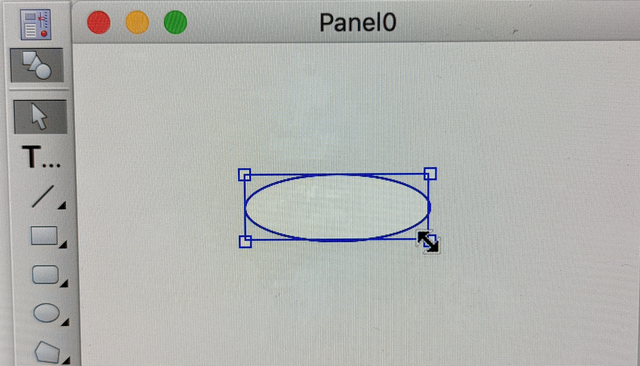Rotate: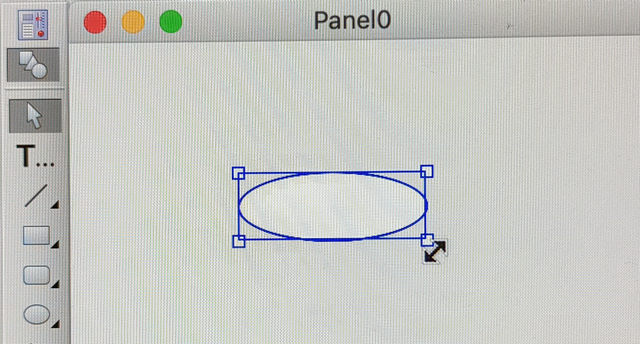You have to move the mouse just outside the handle to get this mode. Less than ideal...

Thanks John !

Indeed, I can now find the rotate arrows in Igor8 and 9 on my MAC.

I guess the trick is to find the "rotate" arrows is to Move the cursor away from the center of the ellipse along the diagonal of the cursor square...

Unfortunately, it seems that even if I used absolute units, the behavior of the "rotation" is not what I expected ...

It seems to resize the ellipse at the same time.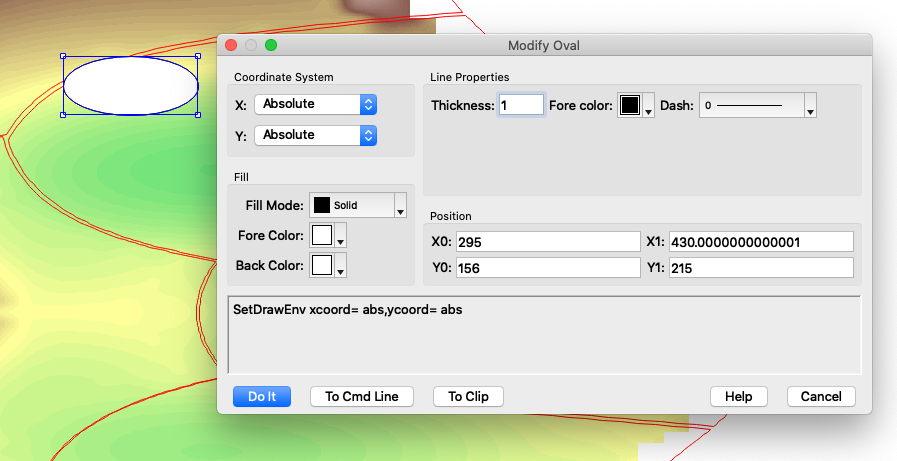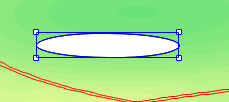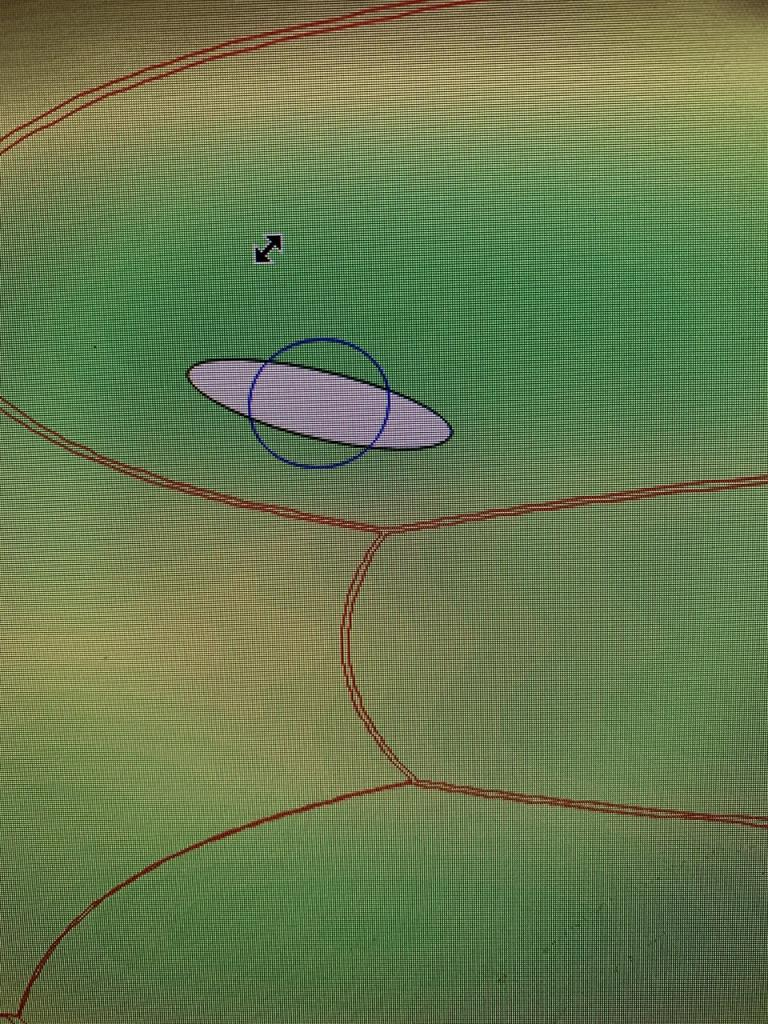My guess is that you have non-isotropic coordinates. That is, the size of a unit in the Y direction is different from the size of a unit in the X direction. That happens commonly with graphs if you draw in relative coordinates or in axis coordinates. The relative coordinates would be isotropic if the window is square.

Thanks Jim for adding this to Igor !

I tested it on a Mac and it works really well for the first ellipse i drew on a test image.

But it looks like behavior reverts to the rotation/distortion if i draw more than one ellipse :(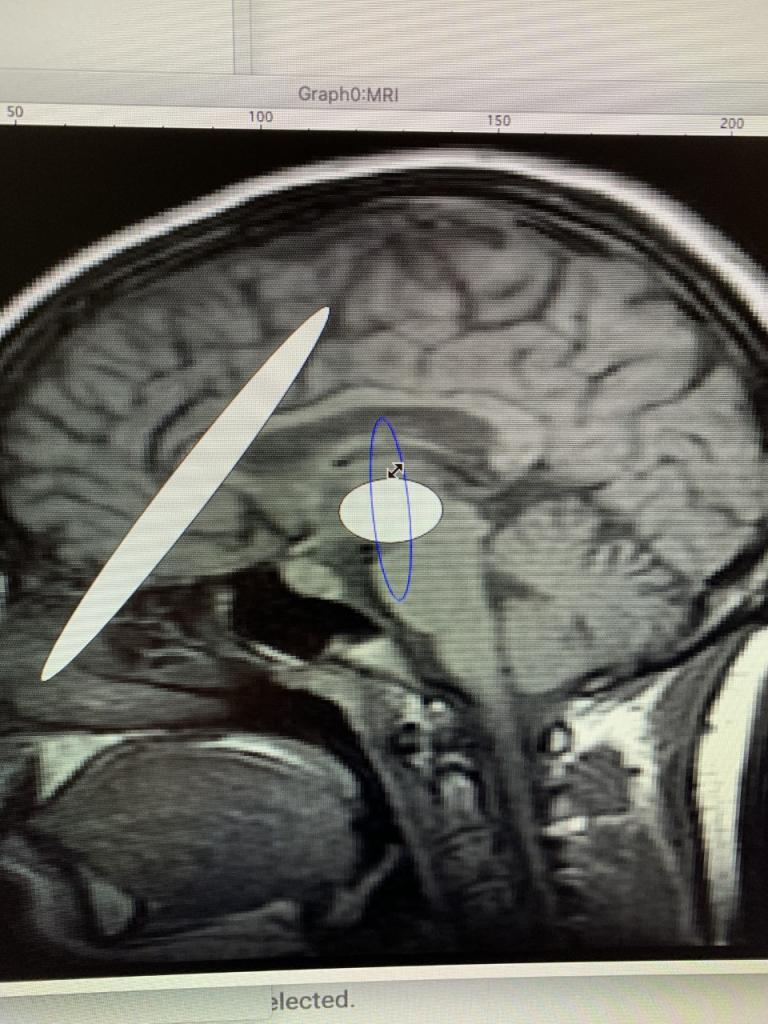To be clear, the rotation in Igor 9.01 isn't *better*, it is just allowed to happen regardless of whether it makes *sense* to rotate in a non-isotropic coordinate system.

Since drawing tools default to the plot relative coordinate system, you're probably drawing in plot relative coordinates. Unless the plot area is perfectly square (plot width = plot height) that's not going to be an isotropic coordinate system.

To make plot relative coordinates isotropic, set the graph width = graph height, either by using auto for one dimension and Aspect = 1 for the other:

ModifyGraph width=0,height={Aspect,1}

When drawing ellipses in axis coordinates (usually the more useful thing to do), use the Plan mode for one dimension with a multiplier of 1:

ModifyGraph width=0, height={Plan,1,left,top}
Window Graph1() : Graph
PauseUpdate; Silent 1       // building window...
Display /W=(702,68,968,349)
AppendImage/T MRI
ModifyImage MRI ctab= {*,*,Grays,0}
ModifyGraph margin(left)=14,margin(bottom)=14,margin(top)=14,margin(right)=14,height={Plan,1,left,top}
ModifyGraph mirror(left)=2,mirror(top)=0
ModifyGraph nticks(left)=6
ModifyGraph minor=1
ModifyGraph fSize=9
ModifyGraph standoff=0
ModifyGraph tkLblRot(left)=90
ModifyGraph btLen=3
ModifyGraph tlOffset=-2
SetAxis/A/R left
ShowTools/A
SetDrawLayer UserFront
SetDrawEnv xcoord= top,ycoord= left
DrawOval 37.5,31.5,83.5,162.5
SetDrawEnv xcoord= top,ycoord= left